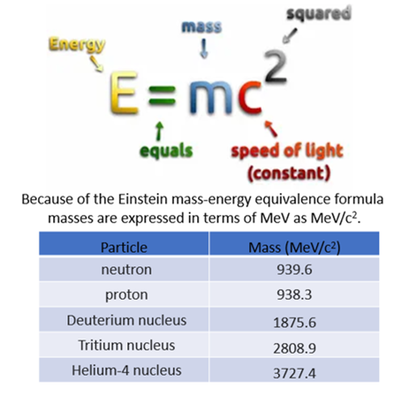Electronvolt (eV) is a unit of energy. An electronvolt is the amount of energy gained by an electron accelerating in an electric potential difference of one volt.
MeV (Mega Electronvolt) = 1 million eV; 1 GeV = 100 MeV; etc.

Particles with different energies interact differently with matter, hence 14 MeV neutrons from fusion produce more radiation damage in material than 2 MeV neutrons from fission.

According to the famous Einstein mass-energy equivalence formula, masses are also expressed using MeV. This is the basis of the conversion of mass into energy in fusion reactors as can be calculated by adding Deuterium and Tritium masses and subtracting the Helium + Neutron mass giving the 17.5 MeV energy gain in D-T fusion.